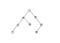# What is DFS ?graph data structure

1 -> 2 -> 4 -> 7 -> 5 -> 3 -> 6 -> 8

# Maze Creation

`// for simplicity stack can be implemented as an arraystack = []// take the initial node and mark it as visited, add it to the stackstart.visited = Truestack.append(start)// do the operation until the stack become emptywhile len(stack) > 0:    //pop the last node from the stack and make it as current    current = stack.pop(0)    if current.has_unvisited_neighbors():        // current node has unvisited neighbors get a random one        neighbor = current.get_random_neighbor()        // push the current node to stack        stack.append(current)        // remove the wall between the current and neighboring node        remove_wall_between(current, neighbor)        // moving to neighboring node and adding it to stack        neighbor.visited = True        stack.append(neighbor)`
`{     row: i,     col: j,     // borders     top: true,     left: true,     bottom: true,     right: true,     // check if already visited     visited: false}`
`export function createMaze(grid) { // stack DS to track the current node const stack = []// starting with first node and mark it as visited stack.push(grid) grid.visited = truewhile (stack.length > 0) {  // taking the last item visited in the stack and get all their nighbours  const current = stack.pop()  const neighbours = getAllNeighbours(current, grid)// proceed only if there is atleast one neighbour  if (neighbours.length > 0) {   // as the current node has a nighbour it is added to the stack   stack.push(current)   // get a radom neighbour from the neighbours list   const neighbour = getRandomNeighbour(neighbours)   removeWall(current, neighbour)// mark the neighbour as visited   neighbour.visited = true   stack.push(neighbour)  } }}`
`// get all the neighbour for maze creation// visited neighbour is not valid herefunction getAllNeighbours(current, grid) { const neighbours = [] const [row, col ] = getPos(current)if (row > 0 && !grid[row - 1][col].visited)// top  neighbours.push(grid[row - 1][col])if (row < ROWS - 1 && !grid[row + 1][col].visited)// bottom  neighbours.push(grid[row + 1][col])  if (col > 0 && !grid[row][col - 1].visited)// left  neighbours.push(grid[row][col - 1])  if (col < COLS - 1 && !grid[row][col + 1].visited)// right  neighbours.push(grid[row][col + 1])return neighbours}`
`removeWall(current, neighbour) {// removing the wall between the current and the considered neighbour   if (current.row > neighbour.row) {    current.top = false    neighbour.bottom = false   }   else if (current.row < neighbour.row) {    current.bottom = false    neighbour.top = false   }   else if (current.col > neighbour.col) {    current.left = false    neighbour.right = false   }   else {    current.right = false    neighbour.left = false   }}`
`// get a random neighbour from the list of neighboursfunction getRandomNeighbour(neighbours) { const index = Math.floor(Math.random() * neighbours.length) return neighbours[index]}`

Thanks for reading. Enjoy your day

Web Developer all the way from India

## More from Ganapathy P T

Web Developer all the way from India